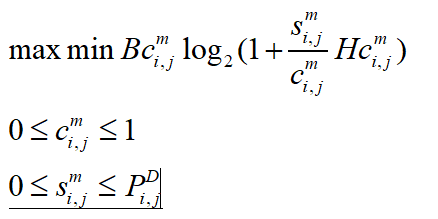# The corresponding function of the max-min problem

Hi.I writting my optimization problem in cvx and running that but i had a error . My question is a max-min problem. I don’t know the corresponding function of the max-min problem in CVX. Please if it is possible help me.cvx_begin

``````        variables sm_h(NUM_DUG,NUM_RB)  T_h(NUM_DUG,NUM_RB)
expressions term1
objfunc=cvx(zeros(NUM_DUG,NUM_RB));
obj2=cvx(zeros(NUM_DUG));
t_ave=0;
for i=1:NUM_DUG
for j=1:NUM_RB
objfunc(i,j)=BW*(-rel_entr(T_h(i,j),sm_h(i,j)*H_D_yong(i,j))/log(2));
obj2(i)=obj2(i)+objfunc(i,j);
end
end

maximize minmize obj2
subject to
term1=0;
for i=1:NUM_DUG
for j=1:NUM_RB
term1(i,j)=BW*(-rel_entr(T_h(i,j),sm_h(i,j)*H_D_yong(i,j))/log(2));
end
end
Size_VI/100000-term1<=0;

T_h>=0;
T_h<=1;
sm_h>=0;
sm_h<=Pt_Dm;
``````

cvx_end

The error message is as follows:

Wrong using of maximize (line 14)
Error: It is not a MATLAB expression.

Error in cvx_cankao1_830 (line 42)
maximize minmize obj2%+penalty*s

Error in main_cankao1_830 (line 103)
cvx_cankao1_830;

Assume you want to do

max min f_i(x)

then you have to do

max s
st f_i(x) >= s

is my guess.